Next: 3.3.1 Krylov Subspace Approximate Up: 3 Simulation of Single Previous: 3.2 Tunneling: a Stochastic

# 3.3 Master Equation Method

The ME method tries to solve (3.12). The main problem of the ME method is to obtain the set of relevant states the circuit under investigation can occupy. The states and their transition rates bring about the circuit describing differential equations which may be written in matrix form for n states as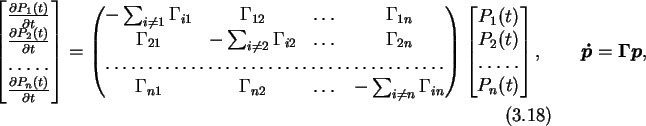where p is the vector of state probabilities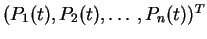. SET circuits with islands have an infinite number of states, because the number of excess electrons is unbound. Clearly higher numbers of excess carriers are due to the Coulomb blockade exponentially suppressed and become more and more unlikely. But it is almost impossible to filter out a priori the most likely states from an arbitrary circuit. The most sensible approach is an adaptive scheme where one starts with the initial state, calculates rates for all possible transitions and uses these rates as a zero-order estimate for the state probability. High transition rates will lead in general to a state with high probability and vice versa. If the rate falls below a threshold the state will not be considered. Then the ME is solved with all states that passed the threshold test. In the next iterations new states will join the set of relevant states and the circuit will be described better and better. This iterative method may be pictured as a journey in state space. Starting at the set of states already found, which is for the first iteration only the initial state, one step is made at a time starting from every state of the set. These steps may lead to states that are already in the set, or to new states. The rate to the new state determines if it should be taken in the set of relevant states or not.

The stationary case of (3.18),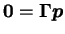, is a system of linear equations which may be solved by a multitude of numerical algorithms . The transient case which is a system of ordinary linear first order homogeneous differential equations, may be solved either by formally integrating (3.18)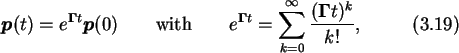and calculating the exponential of a matrix, or by solving the system of differential equations without explicitly forming the exponential of the transition rate matrix. For taking the exponential of a matrix the rational  Padé approximation is one of the better algorithms available  . Nevertheless, a major disadvantage of Padé approximants is that they are accurate only near the origin and so should not be used when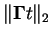is large. One can assure the smallness of the norm by making t accordingly small. This means that the time discretisation might become inconveniently small. A description of rational Padé approximation is given in Appendix G. For the solution of ordinary differential equations a wide variety of methods exist, such as Euler, Taylor series, Runge-Kutta, single-step, and multi-step methods  . Most methods experience difficulty if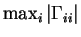, the largest exit rate from any state, and t, the time interval in which the solution is required, are large. Only the relatively novel approach of using Krylov subspaces is promising over a large range of parameters, since this method isolates the eigenvalues which dominate the matrix exponential. Therefore we outline the basic Krylov subspace method in more detail.Next: 3.3.1 Krylov Subspace Approximate Up: 3 Simulation of Single Previous: 3.2 Tunneling: a Stochastic

Christoph Wasshuber